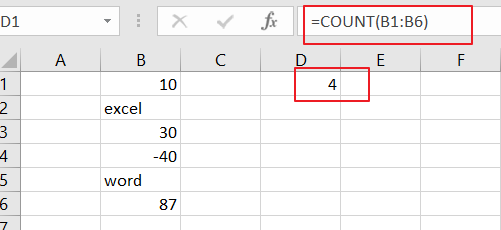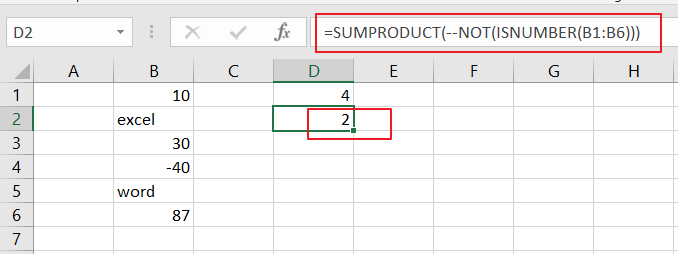# How to Count Cells that Contain only numbers in Excel

This post will guide you how to count the number of cells that only contain numbers within a range of cells using a formula in Excel 2013/2016.

## Count Number of Cells that Contain Numbers

Assuming that you have a data list in the range of cells B1:B6, and you want count the number of cells containing only numbers, and write the result in cell D1.You can use a formula based on the COUNT function  Like this:

=COUNT (B1:B6)

The Syntax of COUNT function is as below:

=COUNT(value1,value2…)

Note: Value1 is reequired, which can be a cell range, a row or a column.

You would see that the above formula COUNT function should be count number of cells contain only number within the range B1:B6.If you want to count the number of cells that does not contain numbers, and you can use a formula based on the SUMPRODUCT function and the ISNUMBER function, like this:

=SUMPRODUCT(–NOT(ISNUMBER(B1:B6)))

Let’s see that how this formula works:

=ISNUMBER(B1:B6)

The ISNUMBER function can be used to search number in the range B1:B6 and returns TRUE or FALSE values. The above function would return an array result;

{TRUE;FALSE;TRUE;TRUE;FALSE;TRUE}

=NOT(ISNUMBER(B1:B6))

The NOT function will convert the above array result in reverse. And the result still is an array like this:

{FALSE;TRUE;FALSE;FALSE;TRUE;FALSE}

Then you need to use double negative operator convert the above result array into 1 and 0, like this:

{0;1;0;0;1;0}

The SUMPRODUCT function will be used to add the array and return final result.### Related Functions

• Excel SUMPRODUCT function
The Excel SUMPRODUCT function multiplies corresponding components in the given one or more arrays or ranges, and returns the sum of those products. The syntax of the SUMPRODUCT function is as below:= SUMPRODUCT (array1,[array2],…)…
• Excel ISNUMBER function
The Excel ISNUMBER function returns TRUE if the value in a cell is a numeric value, otherwise it will return FALSE.The syntax of the ISNUMBER function is as below:= ISNUMBER (value)…
• Excel COUNT function
The Excel COUNT function counts the number of cells that contain numbers, and counts numbers within the list of arguments. It returns a numeric value that indicate the number of cells that contain numbers in a range…
• Excel NOT function
The Excel NOT function returns the opposite of a given logical or Boolean value. For example, if you supplied with the value TRUE, the NOT function will return FALSE; If you supplied with the value FALSE, and the NOT function will TRUE. The syntax of the NOT function is as below:=NOT(logical)…